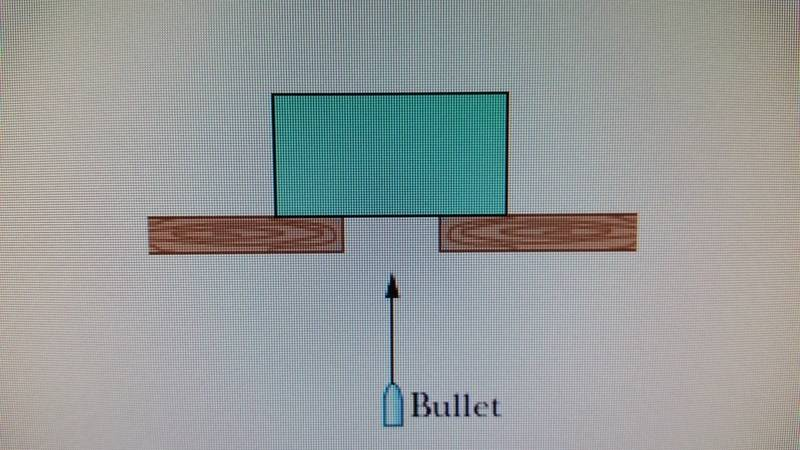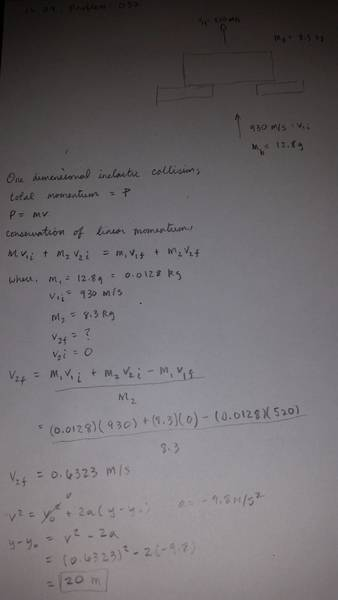# One dimensional inelastic collision; bullet through block.

## Homework Statement

In the figure here, a 12.8 g bullet moving directly upward at 930 m/s strikes and passes through the center of mass of a 8.3 kg block initially at rest. The bullet emerges from the block moving directly upward at 520 m/s. To what maximum height does the block then rise above its initial position?## Homework Equations

m1v1i + m2v2i = m1v1f + m2v2f

## The Attempt at a Solutionnot sure where i went wrong with this one.

SammyS
Staff Emeritus
Homework Helper
Gold Member

## Homework Statement

In the figure here, a 12.8 g bullet moving directly upward at 930 m/s strikes and passes through the center of mass of a 8.3 kg block initially at rest. The bullet emerges from the block moving directly upward at 520 m/s. To what maximum height does the block then rise above its initial position?

[ IMG]http://i1164.photobucket.com/albums/q562/falsovero/20150707_211326_zpsglwxmosy.jpg[/PLAIN]

## Homework Equations

m1v1i + m2v2i = m1v1f + m2v2f

## The Attempt at a Solution

[ IMG]http://i1164.photobucket.com/albums/q562/falsovero/20150707_210809_zpsywi6ntir.jpg[/PLAIN]
not sure where i went wrong with this one.
Velocity for block is correct.

Bad algebra in solving for y-y0 .

•J-dizzal
Nathanael
Homework Helper
Instead of y-y0 I will call it h and instead of a, I will call it g.

You wrote the equation v2=2gh... good so far... but then you wrote h=v2-2g•J-dizzal
Instead of y-y0 I will call it h and instead of a, I will call it g.

You wrote the equation v2=2gh... good so far... but then you wrote h=v2-2gI need to take an algebra class, again.

why is g positive in this problem? because the bullet is fired straight up and so the accerleration of g is -9.8m/s/s. I can see it works out in the equation for it to be positive, i just dont know the reason why.

Last edited:
Thank you

SammyS
Staff Emeritus
Homework Helper
Gold Member
is g positive here? or would it be negative because it in the negative y direction?
So I'm assuming that you resolved the above question, because of your following post.
Thank you
It may be helpful to you and us, if you could learn to use the edit feature and/or the delete feature to alter or to remove a post like Post #5.

So I'm assuming that you resolved the above question, because of your following post.

It may be helpful to you and us, if you could learn to use the edit feature and/or the delete feature to alter or to remove a post like Post #5.
Sure, i didnt know about the delete feature. I'll edit it above.

Nathanael
Homework Helper
why is g positive in this problem? because the bullet is fired straight up and so the accerleration of g is -9.8m/s/s. I can see it works out in the equation for it to be positive, i just dont know the reason why.
Because it is really Vf (which you called V) that is zero, and the V0 is what is 0.6323. So g would be negative but you would also have to subtract V02 over to the other side and then the negative sign would cancel out.

Because it is really Vf (which you called V) that is zero, and the V0 is what is 0.6323. So g would be negative but you would also have to subtract V02 over to the other side and then the negative sign would cancel out.
Ok, i think i get it now because the problem is treated as two problems and the v_f i got from the first problem becomes v0 in the next. Thanks.